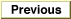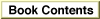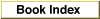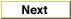# Legacy DocumentImportant: The information in this document is obsolete and should not be used for new development.

Inside Macintosh: QuickDraw GX Environment and Utilities /
Chapter 8 - QuickDraw GX Mathematics / QuickDraw GX Mathematics Reference
Mathematical Functions / Vector Operations

### VectorMultiplyDivide

You can use the `VectorMultiplyDivide` function to calculate the quotient of the dot product of two vectors and a divisor.

```long *VectorMultiplyDivide(long count, const long *vector1,
long step1, const long *vector2,
long step2, long divisor);
```
`count`
The size of each vector.
`vector1`
A pointer to one of the two vectors.
`step1`
The index increment for the `vector1` vector.
`vector2`
A pointer to the second of two vectors.
`step2`
The index increment for the `vector`2 vector.
`divisor`
The number by which the dot product is to be divided.
function result
The quotient of the dot product of two vectors and a divisor.
##### DESCRIPTION
The `VectorMultiplyDivide` function calculates the quotient of a dot product of parameters `vector1` and `vector2` and a `divisor` parameter. The size of each vector is given by the `count` parameter. The index increment is given by the parameters `step1` and `step2`, respectively. If the `count` parameter is negative, the terms are alternated. This is equivalent to

```WideDivide(VectorMultiply(),divisor)
```